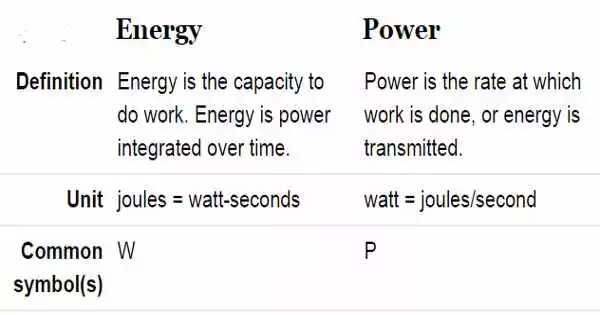Physics

# Difference between Energy and PowerThe capacity of an object to perform work is defined as its energy. Power, on the other hand, denotes the rate at which work is done on an object. The strength required to perform work is referred to as energy, whereas the rate at which work is performed is referred to as power. A joule is the unit of measurement for energy, whereas a watt is the unit of measurement for power.

There is a fine line between how we use the word ‘work,’ in our daily activities, and in science. Work, like our daily lives, involves physical and mental strain, such as dancing, singing, cooking, bathing, washing, learning, and so on. Work, on the other hand, in science refers to the result of a force acting on an object to cause or stop motion, which is measured in joules.

Energy is neither generated nor destroyed; it is only turned from one form to another. On the contrary, power cannot be transformed from one form to another. Conservation of energy is possible. However, power cannot be stored.

Energy and power are two fundamental concepts related to work that are used interchangeably, but they are not the same thing. So, come on in, and let’s talk about the distinctions between them.

Difference between Energy and Power –

ENERGY

• Energy is described as the object’s ability to perform work. It can be measured in terms of Joules, which is equal to watt-seconds. It is indicated by W,
• The term ‘energy’ refers to someone or something’s ability to do work. It denotes a person’s ability to perform work for an extended period of time, i.e. how long one can do a specific activity. As a result, energy has a time component.
• In more technical terms, an object is said to have energy if it is capable of doing work. Furthermore, it can be transferred from one object to another, so that the object doing the work loses energy while the object receiving the work gains it.
• Energy enters our lives in a variety of ways. According to the first law of thermodynamics, we cannot create or destroy energy, but can only convert it from one form to another.

POWER

• Power alludes to the rate at which work is performed upon an object. It is expressed in terms of Watt, which equals Joules per second. It is indicated by P.
• In science, power is a measure that determines the speed at which an object performs work, i.e. how quickly or slowly an object performs work. It is the frequency with which an object performs an activity.
• It is the amount of energy consumed per unit of time, i.e. if an object can transfer more energy in less time, it has more power.
• The time rate or pace at which the work is completed is referred to as power. Power is commonly conveyed or described in “watts,” and energy in “watt-hours.” You can calculate how much energy is consumed in watt-hours by applying power (watts) over time (hours).